# How to Convert Text to Time in Excel

This post will guide you how to convert time string to time in Excel. Assuming that you have a list of text string in your worksheet, and you wish to convert these time string to standard time format, how to do it.

You need to use an formula based on the TIMEVALUE function, the LEFT function, the LEN function and the RIGHT function. Like below:

`=TIMEVALUE(LEFT(B1,LEN(B1)-2)&":"&RIGHT(B1,2))`

Type this formula into a blank cell, and press Enter key on your keyboard to apply it. and drag the AutoFill Handle to other cells to apply this formula.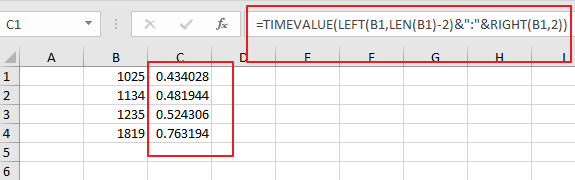Then you need to keep these cells selected, and right click on it, select Format Cells from the context menu list and the Format Cell Dialog will open.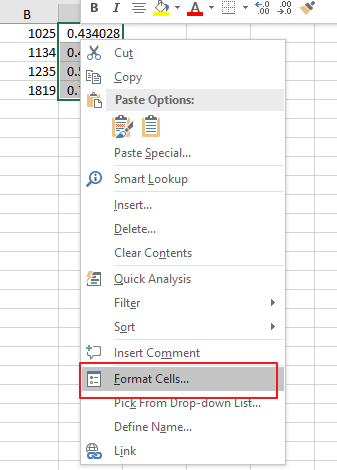Switch to Number tab under Format Cells dialog box, and select Time category from the Category list, and select one time type as you need. click Ok button.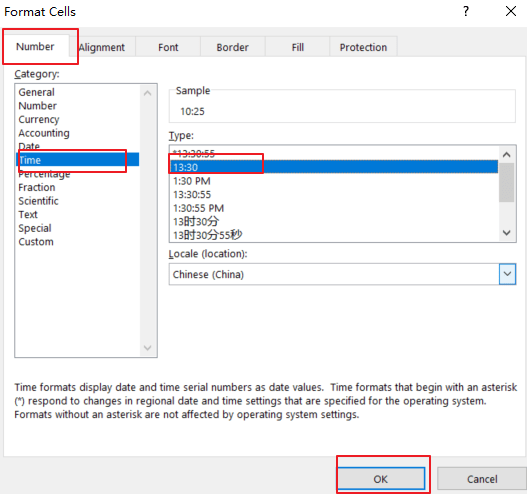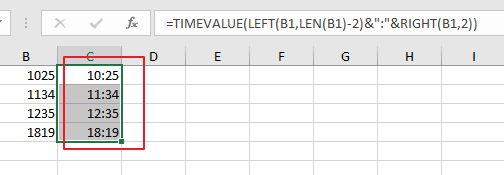If you want to convert date and time string to a data time format in Excel, and you can use the following formula based on the DATE function, the LEFT function, the MID function, the Time Function and the RIGHT function. Like below:

`=DATE(LEFT(B1,4),MID(B1,5,2),MID(B1,7,2))+TIME(MID(B1,10,2),RIGHT(B1,2),0)`

Type this formula into a blank cell and press Enter key on your keyaboard.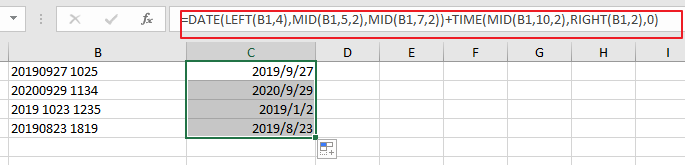Then you need to format those selected cells to a data format as you need.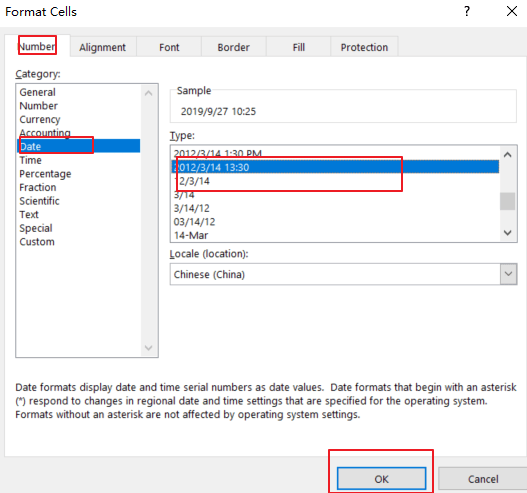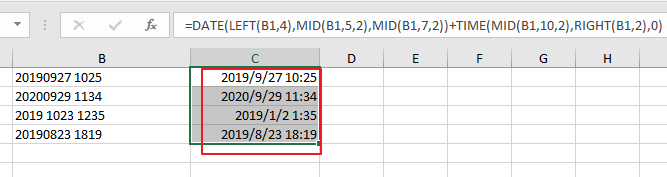### Related Functions

• Excel TIMEVALUE Function
The Excel TIMEVALUE function returns the decimal number of the time represented by a text string. so it will convert a time represented by a text string into an Excel time value.The syntax of the TIMEVALUE function is as below:=TIMEVALUE (time_text)…
• Excel MID function
The Excel MID function returns a substring from a text string at the position that you specify.The syntax of the MID function is as below:= MID (text, start_num, num_chars)…
• Excel LEFT function
The Excel LEFT function returns a substring (a specified number of the characters) from a text string, starting from the leftmost character.The LEFT function is a build-in function in Microsoft Excel and it is categorized as a Text Function.The syntax of the LEFT function is as below:= LEFT(text,[num_chars])…
• Excel RIGHT function
The Excel RIGHT function returns a substring (a specified number of the characters) from a text string, starting from the rightmost character.The syntax of the RIGHT function is as below:= RIGHT (text,[num_chars])…
• Excel TIME function
The Excel TIME function returns a date in serial number format for a particular time (the hour, minute and second).The syntax of the TIME function is as below:= TIME(hour, minute, second)…
Related Posts

How to Extract Time From A Date And Time in Excel

This post will guide you how to extract time portion from a date and time in Excel. How do I remove date on Date/Time Stamp with a formula in Excel. How to remove date from a date and time value ...

How to Convert Military Time to Standard Time in Excel

This post will guide you how to convert military time to standard time with a formula in Excel. How do I convert from military time 17:29:30 to regular time 5:29:30PM in Excel.  How do I Convert Standard time to military ...

How to Remove Prefix and Suffix in Given Cells in Excel

This post will guide you how to remove suffix form a range of cells in Excel. How do I remove prefix from text string in cells with a formula in Excel. Remove Prefix of Cells Remove Suffix of Cells Assuming ...

This post will guide you how to extract email address from a text string in Excel. How do I use a formula to extract email address in Excel. How to extract email address from text string with VBA Macro in ...

Extract Time or Hour Only from a Date and Time in Excel

This post will guide you how to extract time from a data and time value with a formula in Excel. How do I extract time from a date-time number in Excel 2013/2016. How to extract hour only from the date ...

Adding Hours, Minutes, or Seconds to a Date and Time in Excel

This post will guide you how to add hours, minutes, or seconds to a given date and time in a cell in Excel. How do I add hours, minutes or seconds to a time with a formula in Excel. How ...

Extract First or Last N Characters

This post will guide you how to extract the first or last n characters from a text string in one cell in Excel. How do I get the first or last N characters from a string using an Excel formula. ...

Insert The File Path and Filename into Cell

This post will guide you how to insert the file path and filename into a cell in Excel. Or how to add a file path only in a specified cell with a formula in Excel. Or how to add the ...

Extract Part of Text String

This post will guide you how to extract part of text string from a cell in Excel. How do I extract a word from a text string in Excel. How to extract a substring from a text string in a ...

Removing Salutation from Name

This post will guide you how to remove salutations from names in the range of cells in excel. How do I remove the salutation from the names cells with formula in Excel. For example, if you have name salutations and ...

Sidebar